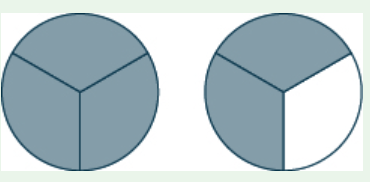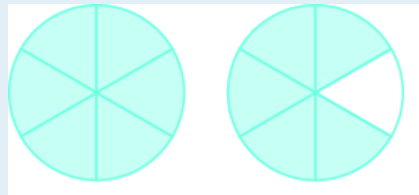Comparing Fractions
Equivalent Fractions
Subtracting Fractions
Unit Fractions
Identify the fraction
100

Which fraction is greater?

A) 3/8

B) 3/9

A) 3/8

100

1/2 = ? /10

5

100

1/4 + 3/4 =

4/4 or 1 whole

100

11/8 - 2/8 =

9/8 or 1 1/8

100

Decompose 7/8 as a SUM of unit fractions

1/8 + 1/8 + 1/8 + 1/8 + 1/8 + 1/8 + 1/8

100

Draw 2/4

200

Which fraction is smaller?

A) 5/10

B) 5/16

B) 5/16

200

3/4 = 12/?

16

200

1/6 + 2/6 =

3/6

200

8/6 - ___ = 1/6

7/6

200

Decompose 11/8 as a Sum of unit fractions

1/8 + 1/8 + 1/8 + 1/8 + 1/8 + 1/8 + 1/8 + 1/8 + 1/8 + 1/8 + 1/8 =

200

Draw 5/4

300

Is 9/20 greater or less than 1/2?

A) greater than

B) less than

B) Less than

300

7/8 = 14/?

16

300

3 5/8 + 3/8 =

4 or 3 8/8

300

1 3/4 - 2/4 =

1 1/4

300

1/3 + 1/3 + 1/3 + 1/3 + 1/3

Draw this in a model.300

Draw 1 1/4

400

Which fraction is greater?

A) 7/11

B) 11/7

C) 7/1

C) 7/1

400

18/20 = 9/?

10

400

4/11 + ____ = 1

7/11

400

9/8 + 2/8

11/8 or 1 3/8

400

Decompose 4/5 into two fractions

2/5 + 2/5 or 1/5 +3/5

400

Draw 7/12

500

Which fraction is smaller?

A) 5/9

D) 8/11

A) 5/9

500

64/80 = ?/10

8

500

Val's construction team was supposed to build a frame 7/10 meters long, but they ended up building the frame 2/10 too long. How long was the frame Val's team built?

9/10 meters long

500

Ruth had 7/8 block of cheese she used to make cheese dip. She used 2/8 blocks of cheese. How much does she have now?

5/8

500Write this as the sum of unit fractions and as a mixed number and improper fraction.

1/6 + 1/6 + 1/6 + 1/6 + 1/6 + 1/6 + 1/6 + 1/6 + 1/6 + 1/6 + 1/6

1 5/6

11/6

500

Draw 3 2/4

Click to zoom